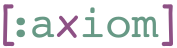## 1    Introduction

This is a small graph library intended to provide topological sort.

In this library, graphs are represented as maps from edges to sets of target edges. For example, the map `{:a #{:b :c} :b #{c:}}` represents a graph with three nodes: `:a`, `:b` and `:c`, where `:a` is connected to both `:b` and `:c`, and `:b` is connected to `:c`.

## 2    merge-graph: Merging Two Graphs

`merge-graph` merges two graphs by adding the edges of the one to the other

``(merge-graph {} {:a #{:b}}) => {:a #{:b}}``

Disjoint graphs are merged by simply merging the maps.

``(merge-graph {:a #{:b}} {:c #{:d}}) => {:a #{:b} :c #{:d}}``

When the two graphs share nodes, their destination sets are merged.

``(merge-graph {:a #{:b :c}} {:a #{:c :d}}) => {:a #{:b :c :d}}``

## 3    invert: Invert the Edges of a DGraph

The inverse of an empty graph is an empty graph.

``(invert {}) => {}``

Each node in the destination set becomes a source edge.

``(invert {:a #{:b :c}}) => {:b #{:a} :c #{:a}}``

When several edges point at the same node, they are placed in the destination set for that node.

``(invert {:a #{:c} :b #{:c}}) => {:c #{:a :b}}``

## 4    souces

`sources` returns the sources in a graph, i.e., the nodes that do not have other nodes pointing to them.

``(sources {:a #{:b :c} :b #{:c}}) => #{:a}``

## 5    toposort: Topological Sort

`toposort` takes a graph and returns a sequence of its nodes that satisfies the partial order defined by the graph.

``(toposort {:a #{:b :c} :b #{:c :d} :c #{:e} :d #{:e}}) => [:a :b :c :d :e]``

### 5.1    Under the Hood

Internally, `toposort` takes the following arguments:

1. `graph`: the graph to be sorted
2. `inv`: its inverse
3. `sources`: a set of its sources

When `sources` is empty, it returns an empty sequence.

``(toposort {} {} #{}) => empty?``

When `sources` has one element and the graph is empty, the element is returned in the sequence.

``(toposort {} {} #{:a}) => [:a]``

When the first source has destinations in the graph, we check if they become sources themselves.

``(toposort {:a #{:b}} {:b #{:a}} #{:a}) => [:a :b]``

However, when the destinations still have other sources (not yet removed from the graph), we do not add them to the sources list.

``````(let [graph {:a #{:b :c} :b #{:c}}]
(toposort graph (invert graph) #{:a})) => [:a :b :c]``````